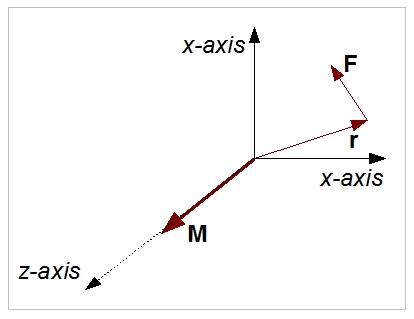# Moment & Couple

## Moment

M = r x F

where:

M : Moment (Nm)
F : Force (N)
r : Arm (m)

The moment of force is a vector quantity that represents the magnitude of force applied to a rotational system at a distance from the axis of rotation.  The SI unit for moment is the Newton metre (Nm).### Explanation

A (force) moment is a measure of the amount of rotation effect due to the working force. The effect depends both on the force and the force arm r. The moment M is a vector quantity, with its axis orthogonal to the plane spawned by F and r.

## Couple

M = d x F

A special case of a moment is a couple. A couple consists of two parallel forces that are equal in magnitude, opposite in sign and do not share a line of action. It does not produce any translation, only rotation. The resultant force of a couple is zero. But, the result of a couple is not zero; it is a pure moment.\### Explanation

A couple is used to describe the rotational effect of two equal forces that do not share a line of action. A couple (or torque) is much used in mechanical engineering. For example, a combustion engine delivers torque at its shaft, which can be used to drive equipment. As stated, a couple is a pure moment and as such can only be destroyed by a couple with a counter effect. Otherwise, the total sum of the forces will not be zero.

### Example

We examine the tail rotor of a helicopter. This rotor is also called the anti torque rotor, as its purpose is to counteract the reaction torque on the fuselage as a result of the applied torque to the main rotor by the engine and transmission. When this reaction torque is not cancelled, the fuselage will rotate. The anti torque rotor functions by introducing a moment, which consists of a thrust vector F which works over the arm 'l' with origin O. This origin O lies on the main rotor shaft. We assume a right angle between the arm 'l' and the tail rotor thrust vector F. The helicopter engine produces a torque of 500 Nm during a hover. The length of 'l' is 5 metres. What force F is needed to prevent the fuselage from spinning?

When we assume a right angle between the arm 'r' and the tail rotor thrust vector F, so the vector products of F and r resolve to the product of their magnitudes. The moment M must counteract the torque of 500Nm; in other words, the sum of the torque and the moment must be zero. This leads to the equation 500 + 5F = 0 -> F = -100N. The minus sign indicates the direction needed to cancel out the effect of the applied engine torque.Will this result in equilibrium in a helicopter? As the sum of moments is zero, the helicopter will not rotate. However, for an object to be in equilibrium, both the sum of moments and the sum of forces in all directions must be zero. We now look at the sum of forces in the plane of rotation, as these are the only relevant forces in this example. These forces are the couple of 500 Nm and the moment of -500Nm. We know that the couple (torque) will not introduce any translational force (by definition).

The sum of the couple is therefore 0. We now look at the anti torque moment of -500 Nm, which uses a force of 100N. As the torque forces are cancelled out, this is the only component left. Conclusion: the sum of all forces is 100 N (500 - 500 + 100).

This force will move the helicopter sidewards! This result should be expected, because a couple (torque) can only be cancelled by another couple, which is not the case with the tail rotor produced moment.

Some manufacturers design their helicopters with the main rotor shaft tilted a number of degrees, in order to counteract the translational force with main rotor thrust. Another solution is to offset the cyclic blade pitch to produce a similar cancelling out thrust vector from the main rotors.

ItemInfoText

Wednesday, January 2, 2019 6:06 PMWhat is couple arm ?

Page: 1

# Do you want to comment this topic?

1: (Book) Cyclic and Collective2: (Book) Principles of Helicopter Flight3: Microsoft FSX Steam Edition4: Logitech Extreme 3D Pro Joystick5: Saitek Pro Flight Rudder Pedals